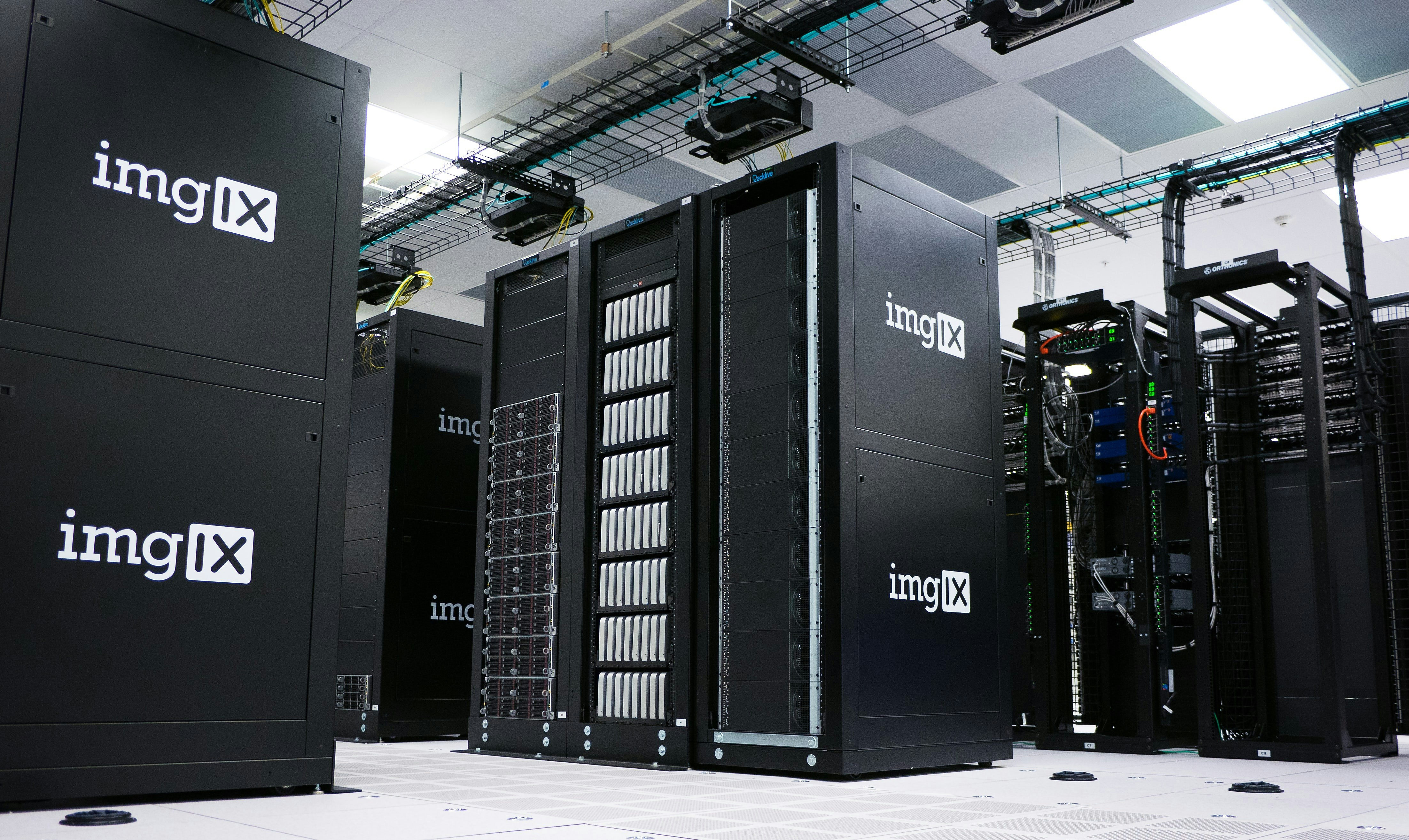Photo by The Roaming Platypus / Unsplash

In the previous part of this series of articles, we made our route through Neural Networks as part of what's known in Machine Learning as Supervised Learning.

We'll continue this series with some of the algorithms used to solve a different kind of problems, that has to do with classification based on intrinsic differents in a data set, differences that an algorithm can infer by itself, without "training" or labeled data, in what's known as Unsupervised Learning.

Let's recap our agenda for this series:

1. Part 1: Supervised Learning: Linear Regression and Logistic Regression
2. Part 2: Supervised Learning: Neural Networks
3. Part 3: Unsupervised Learning: Clustering

Motivation

Unsupervised Learning leaves us to a new field of Machine Learning, where a program doesn't need to be trained prior to solve a problem, but instead, we can give it the means to learn from patterns in the data it parses, using numerical methods and statistics. This unveils a new fascinating world of possibilities and practical uses.

Actually, we can find practical applications of Unsupervised Learning each time we open a Netflix account and it "recommends" series and movies based on our history of choices and preferences. Another example is how e-commerce applications like amazon find products that we might want, even when we're not actively looking for them, but "Amazon" knows it just because of our previous browsing and purchases.

Unsupervised Learning

In Machine Learning, Unsupervised Learning is a category of algorithms that infer a function to describe hidden patterns or structures from "unlabeled" data or data that hasn't been classified by prior observations.

Note that there are no means for accuracy evaluation against a given input, for this kind of algorithm. Its true value resides in inferring boundaries or defining groups of categories more than providing an estimated output for the given input.

Let's see some of the most relevant unsupervised learning algorithms.

Clustering

Clustering or Cluster Analysis is a statistical method consisting on grouping the elements (clusters) in a set of data, based on similarities (in some sense), that is different to other elements in other groups.

Some application of clustering can be found in business and marketing, where is used to research how the general population of consumers is distributed according to their product/service preferences and understand the relationships between these groups.It can also be used to distribute clusters of web servers according to the contents they have in common or shared resources.Photo by imgix / Unsplash

Clustering by itself is not an algorithm, but a type of algorithm. We'll study one of the most used in Machine Learning: K-Means

K-Means Algorithm

This algorithm is a very popular clustering method to partition a set of data into K groups or number of clusters, by finding the optimal mean to all the elements of a specific group, or put in other words, it consists of finding the centroid of each cluster and telling apart what elements belong to what cluster.

Let's then make a formal definition of the K-means algorithm,

Having

• $K$ (number of clusters)
• Training set {$x^{(1)}, x^{(2)}, ..., x^{(m)}$}

$x^{(i)} \in R^n$

Randomly initialize $K$ cluster centroids $\mu_1, \mu_2,...,\mu_K \in R^n$

Repeat {
for $i$ = 1 to $m$
$c^{(i)}$ = index (from 1 to $K$) of cluser centroid closest to $x^{(x)}$

for $k$ = 1 to $K$

Up next, Unsupervised Learning: Anomaly Detection

Thank you so much for reading this article, please take care and I'll see you next time with a third part, we'll continue seeing the algorithms in the Unsupervised Learning category, like Anomaly Detection and Recommender Systems. Stay tuned!.

1. Taken from wikipedia ↩︎